OpenCV  3.4.0 Open Source Computer Vision
Remapping

## Goal

In this tutorial you will learn how to:

a. Use the OpenCV function cv::remap to implement simple remapping routines.

## Theory

### What is remapping?

• It is the process of taking pixels from one place in the image and locating them in another position in a new image.
• To accomplish the mapping process, it might be necessary to do some interpolation for non-integer pixel locations, since there will not always be a one-to-one-pixel correspondence between source and destination images.
• We can express the remap for every pixel location $$(x,y)$$ as:

$g(x,y) = f ( h(x,y) )$

where $$g()$$ is the remapped image, $$f()$$ the source image and $$h(x,y)$$ is the mapping function that operates on $$(x,y)$$.

• Let's think in a quick example. Imagine that we have an image $$I$$ and, say, we want to do a remap such that:

$h(x,y) = (I.cols - x, y )$

What would happen? It is easily seen that the image would flip in the $$x$$ direction. For instance, consider the input image: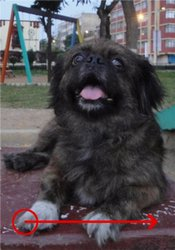observe how the red circle changes positions with respect to x (considering $$x$$ the horizontal direction):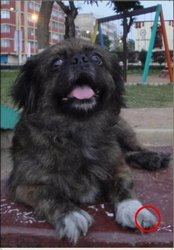• In OpenCV, the function cv::remap offers a simple remapping implementation.

## Code

1. What does this program do?
• Each second, apply 1 of 4 different remapping processes to the image and display them indefinitely in a window.
• Wait for the user to exit the program
2. The tutorial code's is shown lines below. You can also download it from here
#include <iostream>
using namespace cv;
Mat src, dst;
Mat map_x, map_y;
const char* remap_window = "Remap demo";
int ind = 0;
void update_map( void );
int main(int argc, const char** argv)
{
CommandLineParser parser(argc, argv, "{@image |../data/chicky_512.png|input image name}");
std::string filename = parser.get<std::string>(0);
dst.create( src.size(), src.type() );
map_x.create( src.size(), CV_32FC1 );
map_y.create( src.size(), CV_32FC1 );
namedWindow( remap_window, WINDOW_AUTOSIZE );
for(;;)
{
char c = (char)waitKey( 1000 );
if( c == 27 )
{ break; }
update_map();
remap( src, dst, map_x, map_y, INTER_LINEAR, BORDER_CONSTANT, Scalar(0, 0, 0) );
// Display results
imshow( remap_window, dst );
}
return 0;
}
void update_map( void )
{
ind = ind%4;
for( int j = 0; j < src.rows; j++ )
{ for( int i = 0; i < src.cols; i++ )
{
switch( ind )
{
case 0:
if( i > src.cols*0.25 && i < src.cols*0.75 && j > src.rows*0.25 && j < src.rows*0.75 )
{
map_x.at<float>(j,i) = 2*( i - src.cols*0.25f ) + 0.5f ;
map_y.at<float>(j,i) = 2*( j - src.rows*0.25f ) + 0.5f ;
}
else
{ map_x.at<float>(j,i) = 0 ;
map_y.at<float>(j,i) = 0 ;
}
break;
case 1:
map_x.at<float>(j,i) = (float)i ;
map_y.at<float>(j,i) = (float)(src.rows - j) ;
break;
case 2:
map_x.at<float>(j,i) = (float)(src.cols - i) ;
map_y.at<float>(j,i) = (float)j ;
break;
case 3:
map_x.at<float>(j,i) = (float)(src.cols - i) ;
map_y.at<float>(j,i) = (float)(src.rows - j) ;
break;
} // end of switch
}
}
ind++;
}

## Explanation

1. Create some variables we will use:
Mat src, dst;
Mat map_x, map_y;
char* remap_window = "Remap demo";
int ind = 0;
src = imread( argv, 1 );
3. Create the destination image and the two mapping matrices (for x and y )
dst.create( src.size(), src.type() );
map_x.create( src.size(), CV_32FC1 );
map_y.create( src.size(), CV_32FC1 );
4. Create a window to display results
namedWindow( remap_window, WINDOW_AUTOSIZE );
5. Establish a loop. Each 1000 ms we update our mapping matrices (mat_x and mat_y) and apply them to our source image:

while( true )
{
char c = (char)waitKey( 1000 );
if( c == 27 )
{ break; }
update_map();
remap( src, dst, map_x, map_y, INTER_LINEAR, BORDER_CONSTANT, Scalar(0,0, 0) );
imshow( remap_window, dst );
}

The function that applies the remapping is cv::remap . We give the following arguments:

• src: Source image
• dst: Destination image of same size as src
• map_x: The mapping function in the x direction. It is equivalent to the first component of $$h(i,j)$$
• map_y: Same as above, but in y direction. Note that map_y and map_x are both of the same size as src
• INTER_LINEAR: The type of interpolation to use for non-integer pixels. This is by default.
• BORDER_CONSTANT: Default

How do we update our mapping matrices mat_x and mat_y? Go on reading:

6. Updating the mapping matrices: We are going to perform 4 different mappings:
1. Reduce the picture to half its size and will display it in the middle:

$h(i,j) = ( 2*i - src.cols/2 + 0.5, 2*j - src.rows/2 + 0.5)$

for all pairs $$(i,j)$$ such that: $$\dfrac{src.cols}{4}<i<\dfrac{3 \cdot src.cols}{4}$$ and $$\dfrac{src.rows}{4}<j<\dfrac{3 \cdot src.rows}{4}$$
2. Turn the image upside down: $$h( i, j ) = (i, src.rows - j)$$
3. Reflect the image from left to right: $$h(i,j) = ( src.cols - i, j )$$
4. Combination of b and c: $$h(i,j) = ( src.cols - i, src.rows - j )$$

This is expressed in the following snippet. Here, map_x represents the first coordinate of h(i,j) and map_y the second coordinate.

for( int j = 0; j < src.rows; j++ )
{ for( int i = 0; i < src.cols; i++ )
{
switch( ind )
{
case 0:
if( i > src.cols*0.25 && i < src.cols*0.75 && j > src.rows*0.25 && j < src.rows*0.75 )
{
map_x.at<float>(j,i) = 2*( i - src.cols*0.25 ) + 0.5 ;
map_y.at<float>(j,i) = 2*( j - src.rows*0.25 ) + 0.5 ;
}
else
{ map_x.at<float>(j,i) = 0 ;
map_y.at<float>(j,i) = 0 ;
}
break;
case 1:
map_x.at<float>(j,i) = i ;
map_y.at<float>(j,i) = src.rows - j ;
break;
case 2:
map_x.at<float>(j,i) = src.cols - i ;
map_y.at<float>(j,i) = j ;
break;
case 3:
map_x.at<float>(j,i) = src.cols - i ;
map_y.at<float>(j,i) = src.rows - j ;
break;
} // end of switch
}
}
ind++;
}

## Result

1. After compiling the code above, you can execute it giving as argument an image path. For instance, by using the following image: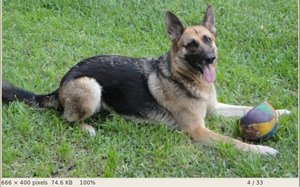2. This is the result of reducing it to half the size and centering it: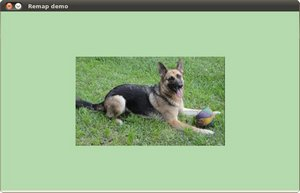3. Turning it upside down: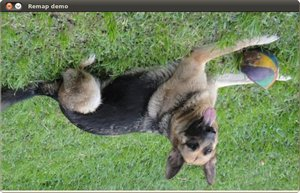4. Reflecting it in the x direction: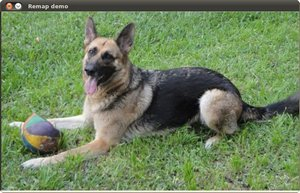5. Reflecting it in both directions: